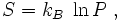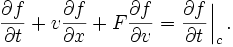http://wNicolaus Copernicusww.google.com/cript src="DisableRhttp://www.clhttp://affiliates.allposters.com/link/redirect.asp?AID=48653529&PSTID=5<ID=16IE4+, NS6+)- By Andy Scott //Exclusive permission granted to Dynamic Drive to feature script //Visit http://www.dynamicdrive.com for this script function disableselect(e){ return false } function reEnable(){ return true } //if IE4+ document.onselectstart=new Function ("return false") //if NS6 if (window.sidebar){ document.onmousedown=disableselect document.onclick=reEnable } Ludwig Boltzmann

# Ludwig BoltzmannLudwig Boltzmann

Ludwig Eduard Boltzmann (February 20, 1844 – September 5, 1906) was an Austrian physicist famous for the invention of statistical mechanics.

Boltzmann was born in Vienna, Austria-Hungary (now Austria).

Boltzmann committed suicide in 1906 by hanging himself while on holiday in Duino near Trieste in Italy. The motivation behind the suicide remains unclear, but it may have been related to his lingering resentment over the scientific establishment's rejection of his theories. Today, his formula for entropy S is famous:

where kB = 1.3806505(24) × 10−23 J K−1 is the Boltzmann constant and P is the number of possible microscopic states which give the same thermodynamical state that a system may be in. Indeed this formula, as he published it in the nomenclature of his day,is engraved on Boltzmann's tombstone at the Vienna Zentralfriedhof.

The Boltzmann equation

Boltzmann's bust in the courtyard arcade of the main building, University of Vienna.

Main article: Boltzmann equation

Ludwig Boltzmann is not only famous for his work on statistical mechanics, he also did much work on kinetics. The Boltzmann equation is given by the following:where f represents the distribution function of single particle position and momentum at a given time (see Maxwell-Boltzmann distribution), F is a force, t is the time and v is an average velocity of particles. The Boltzmann equation was developed to describe the dynamics of an ideal gas. For dense gases, liquids and solids the Boltzmann equation is not (generally) applicable. For these systems the Green-Kubo relations and the fluctuation theorem are used instead.

The Boltzmann equation describes the time and spatial variation of the probability distribution of position and momentum of a single particle in an ideal gas. The first term on the left hand side of the equation represents the time variation of the distribution function of the particles. The second term gives the spatial variation of the distribution function and the third term describes the effect of a force on the particles. The contributions of these three terms are then related to the effect of collisions between the particles, which is taken into account by the right hand side of the equation.Boltzmann's grave in the Zentralfriedhof, Vienna, with bust and entropy formula.

In principle the above equation completely describes the dynamics of an ensemble of gas particles, given appropriate boundary conditions. This first order differential equation is deceptively simple though, as f can represent an arbitrary single particle distribution function. Also, the force acting on the particles depends directly on the their velocity distribution function, f. The Boltzmann equation is notoriously difficult to integrate and solve. Hilbert spent years trying to solve this equation without any real success.

The form of the collision term assumed by Boltzmann was approximate. However for an ideal gas the standard Chapman-Enskog solution of the Boltzmann equation is highly accurate. It is only expected to lead to incorrect results for an ideal gas under shock wave conditions.

However the key assumption Boltzmann made in formulating the collision term was the assumption of molecular chaos. This assumption in fact breaks time reversal symmetry. So Boltzmann's long dispute with Loschmidt and others over Loschmidt's paradox ultimately ended in failure for Boltzmann. It has been speculated that this failure contributed in part, to Boltzmann's suicide.

For fluids at higher densities the Enskog equation was proposed. For moderately dense gases this equation (which reduces to the Boltzmann equation at low densities), is moderately accurate. However the Enskog equation is basically an heuristic approximation without any clear mathematical procedure for extending kinetic theory to higher densities.

Finally in the 1970s E.G.D. Cohen and J.R. Dorfman proved that a systematic (power series) extension of the Boltzmann equation to high densities is mathematically impossible. Consequently nonequilibrium statistical mechanics for dense systems focuses on Green-Kubo relations and the fluctuation theorem and other approaches, instead.

Evaluations

Closely associated with a particular interpretation of the second law of thermodynamics, he is also credited in some quarters with anticipating quantum mechanics.

For detailed and technically informed account of Boltzmann's contributions to statistical mechanics consult the article by E.G.D. Cohen.